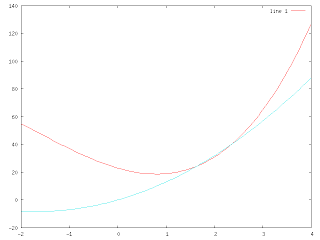## Friday, February 9, 2007

### Plotting in Octave

i've been trying to look for help as far as plotting goes in Octave -
especially plotting a function ... over a range of numbers ...

if you are a beginner like me ... this is what i tried, and it has worked so far for a
2d plot.

to plot a function over a range of numbers ... first create the function ...

function y=f(x) y=x.^3+(5*x.^2)-(10*x)+23; end

and then ..
specify the variable ..
linspace = linear space ...
x = linspace(-2,5);

then just plot it ..

plot(x,f(x)) ...

the result should be something like this ....to add more options to the graph - like grid or title ...
simple do .. options like grid on or title('y vs x') ...

and to plot multiple functions on the same plot ...
trying using the command hold on ...

here's an example ...
given another function

function y=e(x) y = (3*x^.2)+(10*x); end

try

plot(x,f(x))
hold on
plot(x,e(x),'c')use
hold off

to turn off this option
btw , the 'c' in earlier function meant that to plot the graph in the color cyan.

and last but not least ...
to print the graph onto a gif ...

try
gset terminal gif
gset output "something.gif"
replot

when done do
replot close

that's about it ...

here are some really awesome resources for octave ...
http://www.37mm.no/mpy/octave-r.html (great cheat sheet)
http://octave.sourceforge.net/index/plot.html
(more plotting packages like fplot and multiplot, awesome addition to the standard octave library)

and obviously ...
http://www.gnu.org/software/octave/

Octave is a great alternative to Matlab and such ...
i had it installed on my Windows XP laptop on top of Cygwin ,
and usually run it on top of Cygwin X when graphing ...
resources for Cygwin X and how to get its GUI desktop to show up ...
try http://en.wikipedia.org/wiki/Cygwin/X

have fun =)
God bless.

### Thoughts sharings ... and code

so i've decided to post up things/code/tricks that i've learned through different experience and trial and error (especially those that've taken me a while to figure it out ....)
so here we go ...
for my personal blog - http://aggietech.blogspot.com ...
this blog will be purely technical.

daniel